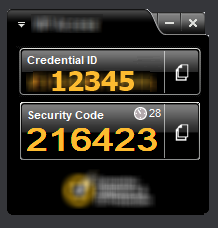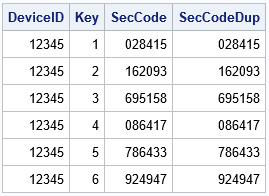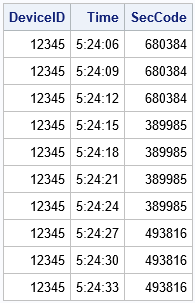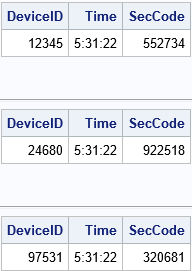In the paper "Tips and Techniques for Using the Random-Number Generators in SAS" (Sarle and Wicklin, 2018), I discussed an example that uses the new STREAMREWIND subroutine in Base SAS 9.4M5. As its name implies, the STREAMREWIND subroutine rewinds a random number stream, essentially resetting the stream to the beginning. I struggled to create a compelling example for the STREAMREWIND routine because using the subroutine "results in dependent streams of numbers" and because "it is usually not necessary in simulation studies" (p. 12). Regarding an application, I asserted that the subroutine "is convenient for testing."

But recently I was thinking about two-factor authentication and realized that I could use the STREAMREWIND subroutine to emulate generating a random token that changes every 30 seconds. I think it is a cool example, and it gives me the opportunity to revisit some of the newer features of random-number generation in SAS, including new generators and random number keys.

### A brief overview of two-factor authentication

I am not an expert on two-factor authentication (TFA), but I use it to access my work computer, my bank accounts, and other sensitive accounts. The main idea behind TFA is that before you can access a secure account, you must authenticate yourself in two ways:

• Provide information that depends on a physical device that you own and that you have previously registered.Most people use a smartphone as the physical device, but it can also be a PC or laptop. If you do an internet search for "two factor authentication tokens," you can find many images like the one on the right. This is the display from a software program that runs on a PC, laptop, or phone. The "Credential ID" field is a long string that is unique to each device. (For simplicity, I've replaced the long string with "12345.") The "Security Code" field displays a pseudorandom number that changes every 30 seconds. The Security Code depends on the device and on the time of day (within a 30-second interval). In the image, you can see a small clock and the number 28, which indicates that the Security Code will be valid for another 28 seconds before a new number is generated.

After you provide a valid username and password, the account challenges you to type in the current Security Code for your registered device. When you submit the Security Code, the remote server checks whether the code is valid for your device and for the current time of day. If so, you can access your account.

### Two-factor random number streams

I love the fact that the Security Code is pseudorandom and yet verifiable. And it occurred to me that I can use the main idea of TFA to demonstrate some of the newer features in the SAS random number generators (RNGs).

Long-time SAS programmers know that each stream is determined by a random number seed. But a newer feature is that you can also set a "key" for a random number stream. For several of the new RNGs, streams that have the same seed but different keys are independent. You can use this fact to emulate the TFA app:

• The Credential ID (which is unique to each device) is the "seed" for an RNG.
• The time of day is the "key" for an RNG. Because the Security Code must be valid for 30 seconds, round the time to the nearest 30-second boundary.
• Usually each call to the RAND function advances the state of the RNG so that the next call to RAND produces a new pseudorandom number. For this application, we want to get the same number for any call within a 30-second period. One way to do this is to reset the random number stream before each call so that RAND always returns the FIRST number in the stream for the (seed, time) combination.

### Using a key to change a random-number stream

Before worrying about using the time of day as the key value, let's look at a simpler program that returns the first pseudorandom number from independent streams that have the same seed but different key values. I will use PROC FCMP to write a function that can be called from the SAS DATA step. Within the DATA step, I set the seed value and use the "Threefry 2" (TF2) RNG. I then call the Rnd6Int function for six different key values.

```proc fcmp outlib=work.TFAFunc.Access; /* this function sets the key of a random-numbers stream and returns the first 6-digit pseudorandom number in that stream */ function Rnd6Int(Key); call stream(Key); /* set the Key for the stream */ call streamrewind(Key); /* rewind stream with this Key */ x = rand("Integer", 0, 999999); /* first 6-digit random number in stream */ return( x ); endsub; quit;   options cmplib=(work.TFAFunc); /* DATA step looks here for unresolved functions */ data Test; DeviceID = 12345; /* ID for some device */ call streaminit('TF2', DeviceID); /* set RNG and seed (once per data step) */ do Key = 1 to 6; SecCode = Rnd6Int(Key); /* get random number from seed and key values */ /* Call the function again. Should produce the same value b/c of STREAMREWIND */ SecCodeDup = Rnd6Int(Key); output; end; keep DeviceID Key SecCode:; format SecCode SecCodeDup Z6.; run;   proc print data=Test noobs; run;```Each key generates a different pseudorandom six-digit integer. Notice that the program calls the Rnd6Int function twice for each seed value. The function returns the same number each time because the random number stream for the (seed, key) combination gets reset by the STREAMREWIND call during each call. Without the STREAMREWIND call, the function would return a different value for each call.

### Using a time value as a key

With a slight modification, the program in the previous section can be made to emulate the program/app that generates a new TFA token every 30 seconds. However, so that we don't have to wait so long, the following program sets the time interval (the DT macro) to 10 seconds instead of 30. Instead of talking about a 30-second interval or a 10-second interval, I will use the term "DT-second interval," where DT can be any time interval.

The program below gets the "key" by looking at the current datetime value and rounding it to the nearest DT-second interval. This value (the RefTime variable) is sent to the Rnd6Int function to generate a pseudorandom Security Code. To demonstrate that the program generates a new Security Code every DT seconds, I call the Rnd6Int function 10 times, waiting 3 seconds between each call. The results are printed below:

```%let DT = 10; /* change the Security Code every DT seconds */   /* The following DATA step takes 30 seconds to run because it performs 10 iterations and waits 3 secs between iterations */ data TFA_Device; keep DeviceID Time SecCode; DeviceID = 12345; call streaminit('TF2', DeviceID); /* set the RNG and seed */ do i = 1 to 10; t = datetime(); /* get the current time */ /* round to the nearest DT seconds and save the "reference time" */ RefTime = round(t, &DT); SecCode = Rnd6Int(RefTime); /* get a random Security Code */ Time = timepart(t); /* output only the time */ call sleep(3, 1); /* delay 3 seconds; unit=1 sec */ output; end; format Time TIME10. SecCode Z6.; run;   proc print data=TFA_Device noobs; var DeviceId Time SecCode; run;```The output shows that the program generated three different Security Codes. Each code is constant for a DT-second period (here, DT=10) and then changes to a new value. For example, when the seconds are in the interval [05, 15), the Security Code has the same value. The Security Code is also constant when the seconds are in the interval [15, 25) and so forth. A program like this emulates the behavior of an app that generates a new pseudorandom Security Code every DT seconds.

### Different seeds for different devices

For TFA, every device has a unique Device ID. Because the Device ID is used to set the random number seed, the pseudorandom numbers that are generated on one device will be different than the numbers generated on another device. The following program uses the Device ID as the seed value for the RNG and the time of day for the key value. I wrapped a macro around the program and called it for three hypothetical values of the Device ID.

```%macro GenerateCode(ID, DT); data GenCode; keep DeviceID Time SecCode; format DeviceID 10. Time TIME10. SecCode Z6.; DeviceID = &ID; call streaminit('TF2', DeviceID); /* set the seed from the device */ t = datetime(); /* look at the current time */ /* round to the nearest DT seconds and save the "reference time" */ RefTime = round(t, &DT); /* round to nearest DT seconds */ SecCode = Rnd6Int(RefTime); /* get a random Security Code */ Time = timepart(t); /* output only the time */ run;   proc print data=GenCode noobs; run; %mend;   /* each device has a unique ID */ %GenerateCode(12345, 30); %GenerateCode(24680, 30); %GenerateCode(97531, 30);```As expected, the program produces different Security Codes for different Device IDs, even though the time (key) value is the same.

### Summary

In summary, you can use features of the SAS random number generators in SAS 9.4M5 to emulate the behavior of a TFA token generator. The SAS program in this article uses the Device ID as the "seed" and the time of day as a "key" to select an independent stream. (Round the time into a certain time interval.) For this application, you don't want the RAND function to advance the state of the RNG, so you can use the STREAMREWIND call to rewind the stream before each call. In this way, you can generate a pseudorandom Security Code that depends on the device and is valid for a certain length of time.

Share